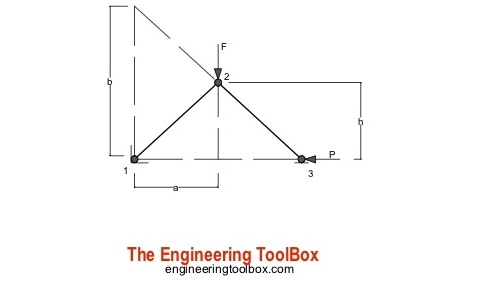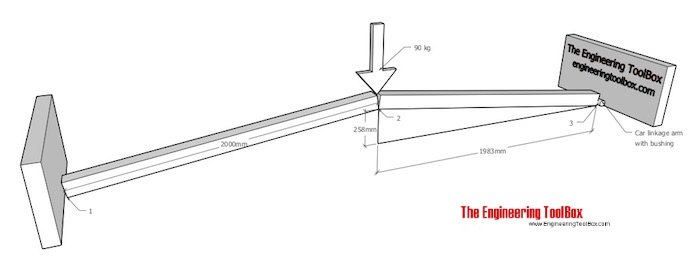Engineering ToolBox - Resources, Tools and Basic Information for Engineering and Design of Technical Applications!

# Toggle Joint

## A toggle joint mechanism can be used to multiply force.The toggle mechanism is made of two arms linked together. Point 1 is fixed, point 3 can move horizontally (as indicated in this principal drawing). The effort force acts vertically in point 2.

The horizontal force acting in point 3 can be calculated for arms with equal or unequal lengths.

### Arms with equal lengths

P = F a / 2 h                            (1)

where

P = horizontal force in point 3 (N, lb)

F = effort force (N, lb)

a = length (m, ft)

h = height (m, ft)

### Arms with unequal lengths

P = F a / b                             (2)

where

b = length (m, ft)

### Example - An Improvised Press for Replacing Car Bushings

A press to remove/refit bushings in car linkage arms can be improvised with two pieces of lumber:The effort force acting on the toggle joint (in point 2) with a person with weight 90 kg can be calculated as

F = (90 kg) (9.81 m/s2)

= 883 N

The force acting on the bush (in point 3) can be calculated as

P = F a / 2 h

= (883 N) (1983 mm) / (2 (258 mm))

= 3393

The force ratio is

Fr = (3393 N) / (883 N)

= 3.8

- the initial effort force is amplified near four times

### Toggle Joint Calculator

This calculator can be used to calculate the resulting force in a toggle joint with arms with equal lengths. The calculator can be used for imperial and metric units as long as the use of units are consistent.

F - force (N, kg, lb)

a - length (m, mm, in, ft)

h - height (m, mm, in, ft)

Note that kg is the SI unit of mass - check the difference between mass and weight (force).

## Related Topics

• Mechanics - Forces, acceleration, displacement, vectors, motion, momentum, energy of objects and more.

## Engineering ToolBox - SketchUp Extension - Online 3D modeling!

Add standard and customized parametric components - like flange beams, lumbers, piping, stairs and more - to your Sketchup model with the Engineering ToolBox - SketchUp Extension - enabled for use with the amazing, fun and free SketchUp Make and SketchUp Pro .Add the Engineering ToolBox extension to your SketchUp from the SketchUp Pro Sketchup Extension Warehouse!

Translate

## Privacy

We don't collect information from our users. Only emails and answers are saved in our archive. Cookies are only used in the browser to improve user experience.

Some of our calculators and applications let you save application data to your local computer. These applications will - due to browser restrictions - send data between your browser and our server. We don't save this data.

## Citation

• Engineering ToolBox, (2018). Toggle Joint. [online] Available at: https://www.engineeringtoolbox.com/toggle-joint-d_2077.html [Accessed Day Mo. Year].

Modify access date.

. .

#### Scientific Online Calculator6 24